## why Maple sometimes uses _C1, _C2, etc.. for const...

I thought in Maple the standard was to use _C1, and _C2, etc... for constants in the solutions returned.

Sometimes Maple mixes _C1 and c in the same result. Is this common, to be expected sometimes and is OK? I noticed this only recently.

I was thinking may be some part of Maple code still was not updated to use _C1 notation? Here is an example

```restart;
pde:=diff(u(x,t),t)+ diff( u(x,t),x )^3 + 6 * u(x,t)* diff(u(x,t),x) = 0;
sol:=pdsolve(pde,u(x,t));
```

which gives

`sol := u(x, t) = -(3/2)*_C1^2+3*(t*_c+x)*_C1-(3/2)*(t*_c+x)^2-(1/6)*_c`

With latest Physics updates  268

## how to make pdsolve return other solutions to nonl...

Is there an option, like AllSolutions used with solve, so that pdsolve would return all solutions to a PDE when it is nonlinear?

I looked at pdsolve help and do not see a HINT that looks like might do this.

For example, this PDE, Maple returns one solution. But Mathematica returns 2 solutions

```restart;
pde:= diff(u(x,t),t) = diff(u(x,t),x\$5)+10*diff(u(x,t),x\$3)*u(x,t)+25*diff(u(x,t),x\$2)*diff(u(x,t),x)+
20*u(x,t)^2*diff(u(x,t),x);
sol:=pdsolve(pde,u(x,t));

#sol := u(x, t) = -12*tanh(176*_C2^5*t+_C2*x+_C1)^2*_C2^2+8*_C2^2
```

But there is another solution

```sol1:=u(x,t)=-(1/2)* _C1^2*(-2 + 3*tanh(x*_C1+ t*_C1^5 + _C2)^2)
pdetest(sol1,pde)
#0
```

Here is another example. Maple returns one solution and Mathematica 7 solutions

```restart;
pde:= diff(u(x,t),t)= u(x,t)*(1-u(x,t))+ diff(u(x,t),x\$2);
sol:=pdsolve(pde,u(x,t));

#sol := u(x, t) = (1/4)*tanh(-5*t*(1/12)+(1/12)*sqrt(6)*x+_C1)^2-
(1/2)*tanh(-5*t*(1/12)+(1/12)*sqrt(6)*x+_C1)+1/4```

But there are other solutions

```pde = D[u[x, t], t] == u[x, t] (1 - u[x, t]) + D[u[x, t], {x, 2}];
DSolve[pde, u[x, t], {x, t}]```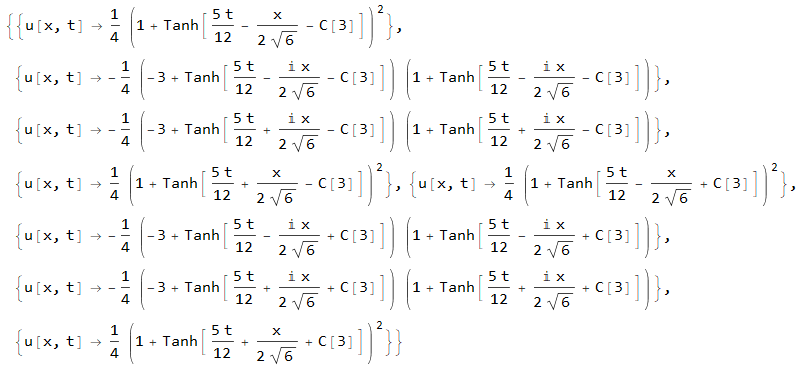I've tested some (not all) of these 7 solutions in Maple using pdetest and Maple agrees they are solutions:

```restart;
pde:= diff(u(x,t),t)= u(x,t)*(1-u(x,t))+ diff(u(x,t),x\$2);
sol:=pdsolve(pde,u(x,t));
with(MmaTranslator);
sol2:=FromMma(`-(1/4) (-3 + Tanh[(5 t)/12 - (I x)/(2 Sqrt) - C]) (1 +
Tanh[(5 t)/12 - (I x)/(2 Sqrt) - C])`);
pdetest(u(x,t)=sol2,pde);
#0```

I tried setting

_AllSolutions:=true

But it had no effect. Is there other options?

## Not return result of an integration...

Hi experts,

I want to compute the following formula using Maple but It returns the formula of integration only.

int(sin(x)/(a*b+a^2*sin(x)^2-d^2*cos(x)^2)(c+cos(x)), x = 0 .. x)

Could you please help to give me some suggestion about how to solve it?

Thank a lot.

## how to view source code in a .maple file (workbook...

I'd like to view the Maple source code in .maple file. I am not able to find how to do this.

I tried commands in 136697-Can-One-Get-The-Source-Code-Back-From

which applies to .mla using the march command. But it did not work for .maple. It gives an error when trying it on .maple file.

I can open the .maple file in the Maple user interface OK using File->Open

but other than documentation, I see no Maple code.

I am looking now at Physics updates file, which can be downloaded as .maple file from

https://maple.cloud/app/5137472255164416

Since most Maple code can be viewed, I assume one can also view the source code in the above.

Any idea how to do this?

## combine/polylog error...

Hello,

I have this error I'm not sure how to solve

restart;

`assuming`([simplify(int(ln(1+x)^3/(x+a), x = 0 .. 1))], [a > 0]);

combine(expand((eval(%, a = I)+eval(%, a = -I))*(1/2)))

What is the precise problem here?

## How does PDEtoos[declare] work?...

I don't quite understand the behavior of PDEtools[declare].  My reading of the documentation is that PDEtools[declare](y(t)) tells Maple that y is a function of t, and therefore y(t) is displayed as y and the derivative of y is displayed as yt.  I did not expect other variables to be similarly affected but apparently they are.  For instance, in the worksheet below, why is the derivative of p displayed as ps?

 > restart;

The normal display of derivatives:

 > diff(y(t),t); diff(p(s),s);Declareas a function of:

 > PDEtools[declare](y(t));> diff(y(t),t);    # this is displayed in subscript notation, as expected diff(p(s),s);    # why is this displayed in subscript notation?>

## power of integers...

In my expresions I have an integer, nx, which actually has values of only +1 and -1 but I do not specify which.

THe results come out as powers of nx, say nx^n, where n is a positive integer.

How do I reduce the expression nx^n,= 1 for n even and nx^n,= nx for n odd?

## pdsolve gives wrong extra arguments: {method = Fou...

Maple 2018.2.1, using Physicsupdates 266.

I undertsand method=Fourier needs boundary conditions to work, but I do not think this error message is right. Compare

```restart;
pde := diff(u(r, theta), r, r)+diff(u(r, theta), theta, theta) = 0;
iv := u(2, theta) = 3*sin(2*theta)+1;
pdsolve([pde,iv], u(r,theta), method = Fourier)```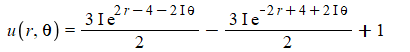With

```restart;
pde := diff(u(r, theta), r, r)+diff(u(r, theta), theta, theta) = 0;
pdsolve(pde, u(r,theta), method = Fourier)```

Error, (in pdsolve/info) wrong extra arguments: {method = Fourier}

pdsolve should return no solution instead. The way it is above, I thought at first I had wrong syntax with the "method = Fourier" settings and I think this error message can be misleading to a user.

## harmless assumptions changes the form of the final...

trying some examples from 209970-Exact-Solutions-For-PDE-And-Boundary--Initial-Conditions-2018

I noticed some answer change when adding assumptions which should not really cause the answer to change. Numerically, both answers seem to be the same. But one is series solution and the other with assumptions added uses integrals.

In wave pde on string where left end at x=0 and adding assumptions x>0,t>0 to pdsolve should produce the same form and it does on some problems but no on others.

Compare

```#example 19, wave pde, on string left end fixed, right end moving, with source, no initial velocity
restart;
pde := diff(u(x, t), t\$2) = 4*diff(u(x, t), x\$2)+(1+t)*x;
bc := u(0,t)=0,u(Pi,t)=sin(t);
ic := u(x,0)=0,eval(diff(u(x,t),t),t=0)=0;

sol:=pdsolve([pde, bc,ic],u(x,t));  #1
sol:=pdsolve([pde, bc,ic],u(x,t)) assuming t>0; #2
sol:=pdsolve([pde, bc,ic],u(x,t)) assuming x>0; #3
sol:=pdsolve([pde, bc,ic],u(x,t)) assuming t>0,x>0;  #4
```

(1,2,3) above give same answer which is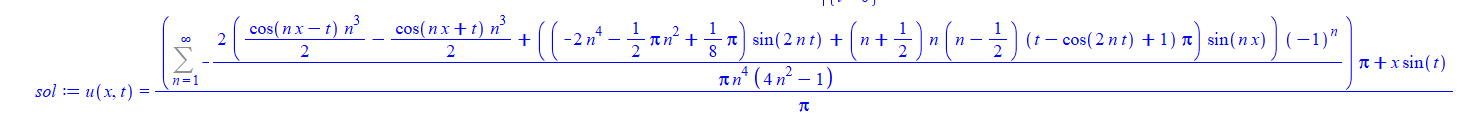but (4) gives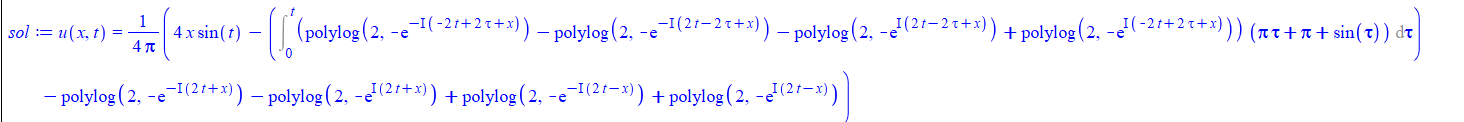Tried random points and numerically they are the same (set the sum to n=100 to evaluate).

my question is why would adding no assuming, or adding t>0 on its own or adding x>0 on its own give same answer, but adding  t>0,x>0 at same time give such a different looking soution.

I typically adding such assumptions to be safe, but here it seems to had some side effect I have not noticed before.

```Physics:-Version();

10:41 hours, version in the MapleCloud: 265, version installed in this computer: not installed

kernelopts(version)
Maple 2018.2, X86 64 WINDOWS, Nov 16 2018, Build ID 1362973
```

## Predict Perfect...

Hi

Initially I tried to find pemutations of 1..9, ie [[1,2,3],[4,5,6],[7,8,9]],[[1,2,4],[3,5,6],[7,8,9]],...etc, But maybe not all of them?

I wonder if someone out there can change my code to reflect the following:

1/According to the pdf excerpt, there are a total of 199 unique sums, the smallest sum is 774 and the largest 2556.(From displaying 280 outcomes, the code got the 774).

2/Show which sums have the greatest probability (specifically, 1566, 1575, 1638, 1656, 1674, 1692, 1755, and 1764, each of which can be shown with effort to have a 3/280, or 1.07%, probability – the calculations to determine this probability is a brute-force computation, and requires enumerating all outcomes of the sample space within a computational software package). We then finally reveal the prediction, which of course is correct..(I don't get it)

predict_perfect.mw

## How to apply a function to two unequal length list...

I want to divide every element of A1 list by every element of list A2. Lists are unequal length.

Example:

A1:= [a1, a2, a3];

A2:= [b1, b2];

Output would be:

[a1/b1, a2/b1, a3/b1, a1/b2, a2/b2, a3/b2]

I managed to do this by this means:

LinearAlgebra:-OuterProductMatrix([a1, a2, a3], [1/b1, 1/b2]); convert(%, list)

My questions are:

1. Is there a more succinct, practical method?
2. How about if I want to use a different operation like say adding or just use a function f?

Thank you all in advanced.

## what is difference between the following syntax f...

I've been using the following syntax to set boundary condition which is a derivative, when passing it to pdsolve. Say we want to set u(r,theta,t) to have insulated boundary conditions at r=1. So the BC will be

For example, to set derivative of u w.r.t. "r" to zero when r=1

eval(  diff(u(r,theta,t),r), r=1) = 0;  #(1)

or using this syntax

D(u)(1, theta, t) = 0;  #(2)

But now I find, on one example below, that the above no longer works.  I have to use this syntax (which I did not know about) for it to work

D*u(1, theta, t) = 0;  #(3)

Has something changed? why when using (3) pdsolve now gives result, but when using (2) or (1) it returns unevaluated? are the three semantically equivalent? when to use which syntax?

I am using Physics updates 265, Latest Maple 2018.2

Here is an example showing the (1,2)  syntax no longer works, but the (3) syntax works

```#articolo example 6.9.2
restart;

#using (1) syntax
pde := diff(u(r, theta, t), t) = (diff(u(r, theta, t), r)+r*(diff(u(r, theta, t), r, r))+(diff(u(r, theta, t), theta, theta))/r)/(25*r);
bc_on_r := eval(diff(u(r,theta,t),r), r=1) = 0;
bc_on_theta:= u(r,0,t)=0, u(r,Pi,t)=0;
ic := u(r,theta,0)=(r-1/3*r^3)*sin(theta);
pdsolve([pde, bc_on_r,bc_on_theta,ic], u(r, theta, t), HINT = boundedseries(r = ))
```

does not solve it.

```restart;

#using (2) syntax
pde := diff(u(r, theta, t), t) = (diff(u(r, theta, t), r)+r*(diff(u(r, theta, t), r, r))+(diff(u(r, theta, t), theta, theta))/r)/(25*r);
bc_on_r := D(u)(1, theta, t) = 0;
bc_on_theta:= u(r,0,t)=0, u(r,Pi,t)=0;
ic := u(r,theta,0)=(r-1/3*r^3)*sin(theta);
pdsolve([pde, bc_on_r,bc_on_theta,ic], u(r, theta, t), HINT = boundedseries(r = ))
```

does not solve it.

```restart;

#using(3) syntax
pde := diff(u(r, theta, t), t) = (diff(u(r, theta, t), r)+r*(diff(u(r, theta, t), r, r))+(diff(u(r, theta, t), theta, theta))/r)/(25*r);
bc_on_r := D*u(1,theta,t)=0;
bc_on_theta:= u(r,0,t)=0, u(r,Pi,t)=0;
ic := u(r,theta,0)=(r-1/3*r^3)*sin(theta);
pdsolve([pde, bc_on_r,bc_on_theta,ic], u(r, theta, t), HINT = boundedseries(r = ))
```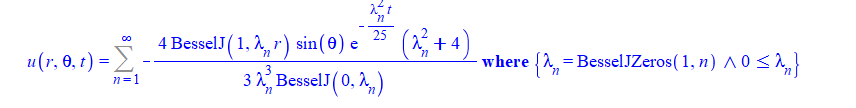I've used syntax (1) before many times and it works. Here is an example where all three syntax work

```pde:=diff(u(x,t),t)=k*diff(u(x,t),x\$2);
bc:=eval(diff(u(x,t),x),x=0)=0,u(L,t)=0;
ic:=u(x,0)=f(x);
sol:=pdsolve([pde,bc,ic],u(x,t));
```

pdsolve gives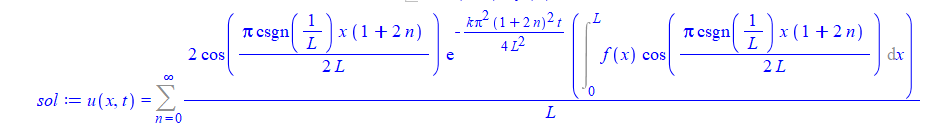Using syntax (2)

```pde:=diff(u(x,t),t)=k*diff(u(x,t),x\$2);
bc:=D(u)(0,t)=0,u(L,t)=0;
ic:=u(x,0)=f(x);
sol:=pdsolve([pde,bc,ic],u(x,t));```gives same answer as (1) and using syntax (3)

```pde:=diff(u(x,t),t)=k*diff(u(x,t),x\$2);
bc:=D*u(0,t)=0,u(L,t);
ic:=u(x,0)=f(x);
sol:=pdsolve([pde,bc,ic],u(x,t));
```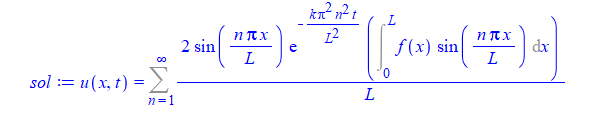The answer also looks like different and simpler, but I assume they are equivalent for now without looking too much into it.

Which syntax should one use as now I am really confused.

It looks like (3) is the one that should be used? Why the others did not work on first example? i.e. pdsolve did not give an answer at all?  And if (1,2,3) syntax are supposed to be equivalent, whysecond example gives slightly different looking answer when using one syntax vs. the other?

And finally to make things more confusing, here is an example where syntax (3) does not work, but syntax 1 and 2 work:

```#articolo example 8.4.3
restart;
pde := diff(u(x, t), t) = (1/20)*(diff(u(x, t), x, x))+t;
bc := u(0, t) = 5, (u(1, t)+ D(u)(1, t)) = 10;
ic:= u(x, 0) = -40*x^2*(1/3)+45*x*(1/2)+5;
pdsolve([pde, bc,ic], u(x, t))
```

```restart;
pde := diff(u(x, t), t) = (1/20)*(diff(u(x, t), x, x))+t;
bc := u(0, t) = 5, (u(1, t)+eval(diff(u(x,t),x),x=1))  = 10;
ic:= u(x, 0) = -40*x^2*(1/3)+45*x*(1/2)+5;
pdsolve([pde, bc,ic], u(x, t))
```

gives same answer. But

```restart;
pde := diff(u(x, t), t) = (1/20)*(diff(u(x, t), x, x))+t;
bc := u(0, t) = 5, (u(1, t)+ D*u(1, t)) = 10;
ic:= u(x, 0) = -40*x^2*(1/3)+45*x*(1/2)+5;
pdsolve([pde, bc,ic], u(x, t))
```

does not work.

Clearly there is something I do not understand between these 3 syntaxes and when to use which.

Using 265 version

```Physics:-Version()

10:41 hours, version in the MapleCloud: 265, version

installed in this computer: not installed```

## Installing packages from the cloud hangs on window...When I try to install (as an example) physics update using Maple 2018.2.1 on windows 10, it keeps hanging in the middle as shown above.

I closed Maple, starting again and tried again, same thing happens. I waited for more than 10 minutes and nothing happens.  I click on the install button in the clouds windows from Maple itself to install it as I always did.

Do others have problem installing this?  I'll try again in few hrs, it might be the Maple server is having some issues but thought to ask.

## Can't solve ODE...

I try to solve ODE with conditions, but it give answer only without conditions:

SOT.mw

Thank you.

## Output what inputs...